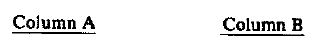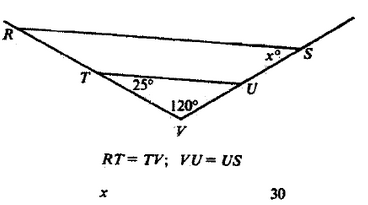# Quantitiative Comparison Angles of a Triangle

The Quantitative Comparison question type on the GRE can be very challenging. Essentially, you are given some information (usually in the form of a sentence, equation, or picture) and then two quantities. You are then asked to determine:

A if the quantity in Column A is greater;

B if the quantity in Column B is greater;

C if the two quantities are equal;

D is the relationship cannot be determined from the information given.

Like most questions on the GRE Quantitative section, the concepts involved in solving this question type are not of themselves very advanced, you just have to be careful in their application. Let’s go through an example problem:First, look at the triangle formed by the points T, U, and V. We are given two angles of this triangle. Remember that the sum of the angles for a triangle will always equal 180°. This means we can subtract the measures of the two marked angles from 180° to find the third angle.

180° – 120° – 25° = 35°

This means that the angle at point U has a measure of 35°.

Now, the key to this problem is that the triangle formed by points R, S, and V has the same angle measurements as the triangle formed by points T, U, and V. The angle at point S is equal to the angle at point U. These two triangles are what are known as similar triangles. Similar triangles are triangles that have equal angles, and whose sides are proportional to each other. In other words, similar triangles will have the same shape, though not necessarily be the same size.

It may seem obvious from the picture that these triangles are similar, but how can we be certain? Generally, you will not want to only rely on the shape of the figure in the picture. Figures on the exam may not be drawn to scale.

We can be certain because of a rule of triangles known as the Side-Angle-Side rule. Here is the rule:

• First, the two triangles must have an equal angle.
• Next, the sides that form the angle on one triangle are equal to the sides that form the angle on the other triangle. Either this, or there is a common ratio between the sides of the two triangles.
• If these two conditions are true then the triangles are similar.

In this case, the two triangles share the 120° angle at point V. Also, the sides forming that angle are in proportion to each other. Notice that line segment RT is equal to segment TV. This means that line segment RV is exactly twice the length of line segment TV. Since line segments VU and US are equal to each other, line segment SV is exactly twice the length of line segment VU. These two triangles pass the Side-Angle-Side rule and must be similar.

Since the triangles are similar, the angle at point S will be equal to the angle at point U. This means that the value of x is 35°. This is greater than 30, which means that the answer is A.

This entry was posted in Example Problems and tagged , , , . Bookmark the permalink.